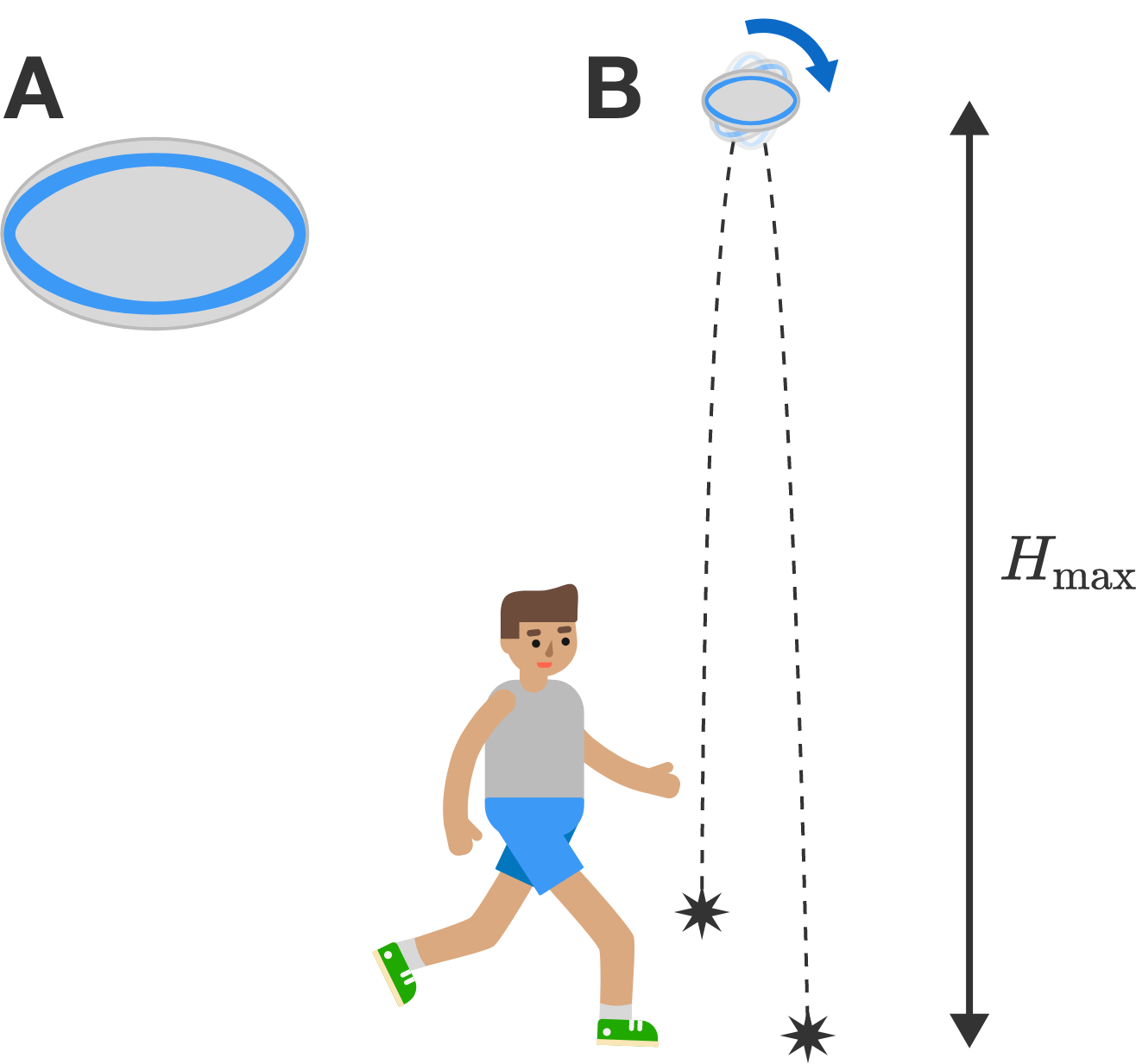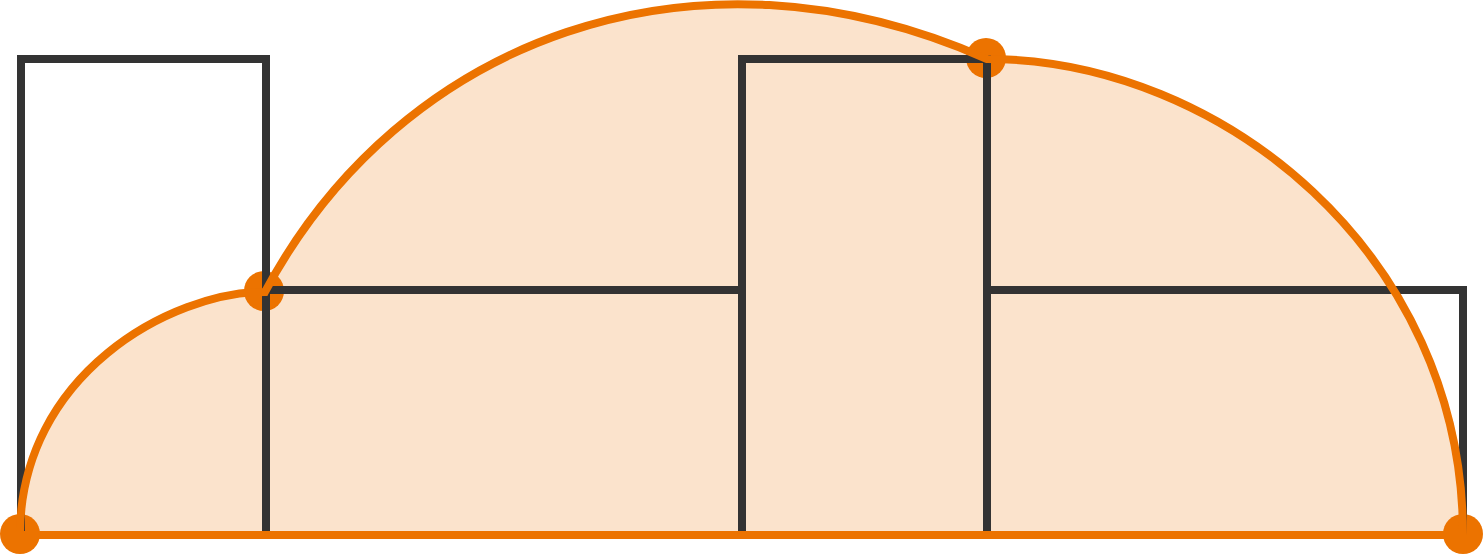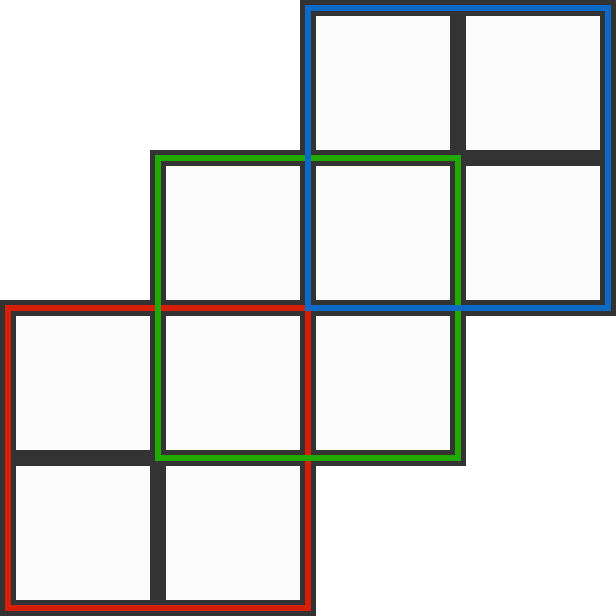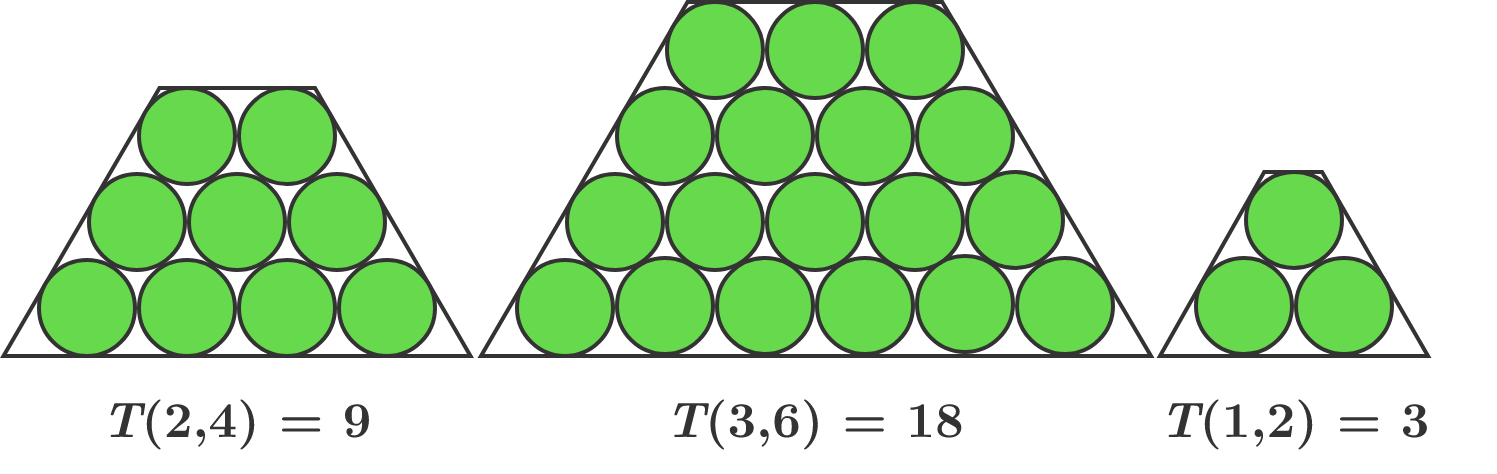# Problems of the Week

Contribute a problem

# 2018-07-09 Intermediate

A rugby ball is kicked such that it spins throughout its entire flight, and reaches a maximum height of $H_\text{max}.$

Is it possible for the ball to bounce higher than $H_\text{max}?$A: A rugby ball is in the shape of an ellipsoid. B: The rugby ball is kicked spinning into the air and achieves its maximum height.

Are there non-zero real numbers $a$, $b,$ and $c$ such that $a+b+c$ and $\frac 1 a + \frac 1 b + \frac 1 c$ are both zero?

A rectangle rolls on a flat surface, and the path of one of its vertices is traced as the rectangle rolls.

If the shorter side of the rectangle is $1$ and the area under the traced path is $3\pi,$ what is the length of the longer side of the rectangle?Place the ten integers 2 through 11 into the ten squares such that the sum of the numbers in each $2 \times 2$ square is the same value, $P.$

What is the maximum possible value of $P?$

$T(x, y)$ is the number of circles in a trapezoidal arrangement such that $x$ is the number of circles in the top row and $y$ is the number of circles in the bottom row. Each row has one fewer circle than the row beneath it does.What is the only number between 10000 and 20000 that cannot be a value of $T(x, y)$ for positive integers $x$ and $y$ such that $x < y?$

×